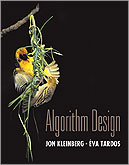Kleinberg and Tardos, Algorithm Design, 2005.

EECS 336: Introduction to Algorithms (Fall 2013)

Required Text: Kleinberg and Tardos, Algorithm Design, 2005.
Discussion/Announcements/Homeworks/etc: on Piazza.

Lectures: Tuesday and Thursday 3:30-4:50 in Tech M345.
Instructor: Jason D. Hartline.
Office Hours: Tuesday, 1:00-2:50, or by appt.; Ford 3-329.

Teaching Assistant: Sam Taggart
Lab Sections: Monday, various times.
Office Hours: Wednesday, 10:30-12:00; Tech B252.

Overview. Algorithm design and analysis is fundamental to all areas of computer science and gives a rigorous framework for the study optimization. This course provides an introduction to algorithm design through a survey of the common algorithm design paradigms of greedy optimization, divide and conquer, dynamic programming, network flows, reductions, and randomized algorithms. Important themes that will be developed in the course include the algorithmic abstraction-design-analysis process and computational tractability (e.g., NP-completeness).

Prerequisites. EECS 212 (Mathematical Foundations of Computer Science) and EECS 214 (Data Structures and Data Management) which cover abstract data types such as stacks, queues, and binary search trees; and discrete mathematics such as recurrence relations, sets, and graphs.

Grading. 50% Homework, 15% Midterm, 25% Final, 10% Participation.

Homework Policy. Homeworks may be done in pairs by students in the same section. Both students must contribute to the solution of all problems. One copy of the assignment should be turned in with the names of both students on it. Both students will receive the same grade. You may consult your text book and course notes when answering homework questions; you must not consult the Internet. Homeworks are assigned in class and due at the beginning of class on Thursday the week after (or as noted). Late homework will be accepted until Monday (at the beginning of your section) and will be marked down by 25% of the grade received.

Tentative Schedule:

Week 1: beginning Sept. 23

• Course overview: computing Fibonacci numbers. (Chapter 1)
• Review of runtime analysis, graphs, and basic graph algorithms. (Chapters 2 and 3)

Week 2: beginning Sept. 30

• Greedy algorithms: interval scheduling. (Chapter 4)
• Greedy algorithms: minimum spanning trees, matroids. (Chapter 4, Matroid Notes)

Week 3: beginning Oct. 7

• Greedy algorithms: shortest paths, MSTs. (Chapter 4)
• Divide and conquer: merge sort, recurrence relations, public key cryptography, repeated squaring (Chapter 5)

Week 4: beginning Oct. 14

• Divide and conquer: integer multiply, convolution, fast Fourier transform (Chapter 5)
• Dynamic programming: memoization, weighted interval scheduling, integer knapsack (Chapter 6)

Week 5: beginning Oct. 21

• Dynamic programming: all pairs shortest paths (Chapter 6)
• Reductions, Network flow, Bipartite Matching (Chapter 7)

Week 6: beginning Oct. 28

• MIDTERM.
• Network flow. (Chapter 7)

Week 7: beginning Nov. 4

• NP and intractability: NP, polynomial time reductions decision problems. (Chapter 8)
• NP and intractability: NP, circuit sat (Chapter 8)

Week 8: beginning Nov. 11

• NP and intractability: circuit sat, 3-sat (Chapter 8)
• NP and intractability: independent set, vertex cover, hamiltonian cycle, TSP.

Week 9: beginning Nov. 18

• Approximation algorithms: TSP, metric TSP, knapsack (Chapter 11)
• Approximation algorithms: pseudo polynomial time, PTAS, knapsack (Chapter 11)

Week 10+: beginning Nov. 25

• Online algorithms: ski-renter, secretary.
• TBA.
• FINAL (cumulative).